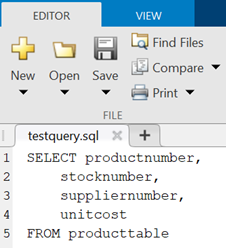## Generate SQL Query and MATLAB Script

You can generate SQL code from an SQL query or create a MATLAB® script by using the Database Explorer app. After defining an SQL query in the SQL Query pane, you can generate the SQL code for running an SQL script. You can also generate a MATLAB script to automate connecting to a database, running an SQL query, and performing data analysis on the imported data.

### Generate SQL Query

To generate SQL code from an SQL query, follow these steps:

1. Connect to a data source and create an SQL query. For details about creating SQL queries, see Create SQL Queries Using Database Explorer App.

2. In the Import section, select Import Data > Generate SQL Query.

3. Save the SQL code to a `.txt` or `.sql` file. The MATLAB Editor opens the saved SQL code file.You can use the SQL code to rebuild a query by using the SQL Query pane by entering the SQL code manually.

Alternatively, you can use the `.sql` file to import data programmatically into MATLAB by using the `executeSQLScript` function.

### Generate MATLAB Script

To generate a MATLAB script that automates connecting to a data source, running an SQL query, and importing the SQL query results, follow these steps:

1. Connect to a data source and create an SQL query. For details about creating SQL queries, see Create SQL Queries Using Database Explorer App.

2. In the Import section, select Import Data > Generate MATLAB Script to display a MATLAB script in the MATLAB Editor. To customize import options before importing data, see Customize Import Options Using Database Explorer App.3. Save the MATLAB script. You can run this script at the command line.

Note

To run the script, enter the database password as an input argument of the `database` function in the script.

For details about editing and running scripts, see Scripts.